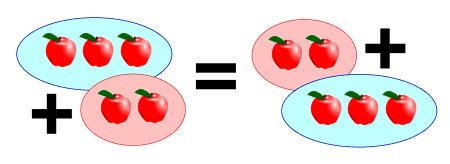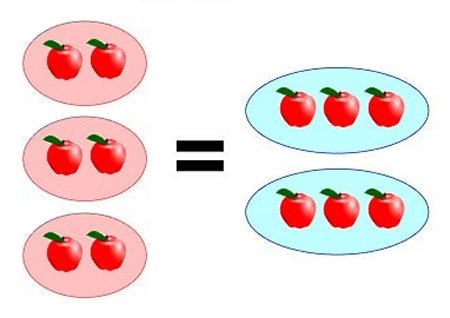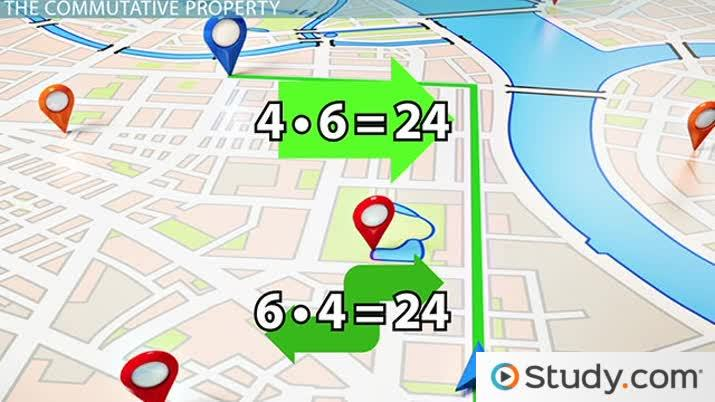# What is the Commutative Property?

Mark Lewis, Jennifer Beddoe
• Author
Mark Lewis

Mark has taught college and university mathematics for over 8 years. He has a PhD in mathematics from Queen's University and previously majored in math and physics at the University of Victoria. He has extensive experience as a private tutor.

• Instructor
Jennifer Beddoe

Jennifer has an MS in Chemistry and a BS in Biological Sciences.

Learn what the commutative property is. Discover examples of the commutative property of multiplication and addition, and examine uses of the commutative property. Updated: 02/17/2022

Show

## What Is the Commutative Property?

In mathematics, a binary operation is a rule that takes two numbers and produces another. Addition and multiplication are both binary operators, since we can add or multiply two numbers together, and the answer is another number.

An operation is said to be commutative if the order of the two inputs values does not change the output value. Symbolically, a binary operation represented by {eq}\star {/eq} is commutative if:

$$a\star b = b\star a$$

Commutativity is one of several properties that mathematical operations like addition, multiplication, subtraction, and division may or may not have. Another property that, at first glance, can be easily confused for commutativity is the associative property. An operation is associative if a series of several operations can be performed in any order, expressed symbolically this means:

$$a\star (b\star c) = (a \star b) \star c$$

In other words, associativity means the placement of brackets does not change the result. The brackets do change the order in which the operations are performed, but the left-to-right order of the inputs does not change, so associativity is different from commutativity.

The distributive property involves both addition and multiplication. Multiplying a number with a sum can be decomposed into the sum of two products, as shown below. Multiplication is said to distribute over addition.

$$a\cdot (b + c) = a\cdot b + a\cdot c$$

The addition of numbers is commutative, meaning that for any numbers {eq}a {/eq} and {eq}b {/eq} we have:

$$a+b = b+a$$The commutative property of addition is easiest to understand with whole-valued positive numbers, but it applies to all numbers, including negative numbers. This means, for example, that:

$$5 + (-2) = (-2) + 5 = 3$$

The addition of a negative value can equally be expressed using the separate operation of subtraction. However, by considering the same example we can see that subtraction is not commutative. Changing the order of the subtraction produces a different, opposite value:

$$5 -2 =3 \qquad\quad 2-5 = -3$$

### Commutative Property of Multiplication

Multiplication of numbers is also commutative: for any numbers {eq}a {/eq} and {eq}b {/eq} we have:

$$a\cdot b = b\cdot a$$

As for addition, the commutative property can be visualized with examples involving whole-valued, positive numbers. For example, the diagram below shows how:

$$3\cdot 2 = 2\cdot 3 =6$$Again, the property does apply to all numbers, including negative numbers. Multiplying a positive with a negative creates a negative value, while multiplying two negatives creates a positive, but in either case the order of the numbers does not change the answer.

The operation of division is equivalent to multiplication by the reciprocal of the second number, so for example:

$$10\div 5 =10 \cdot \frac{1}{5} = 2$$

Multiplication is of course commutative, including when fractional values are involved, but the operation of division is not. For the previous example, we can see that changing the order in the division yields a different answer:

$$5 \div 10 = 5 \cdot \frac{1}{10} = 0.5$$

## The Long Commute

If you commute to school or work, you know that sometimes the traffic can drive you crazy. Maybe, like me, you have four or so different routes you can take to work that all are about the same distance and take roughly the same amount of time (depending on if you catch the lights right or not).

Obviously, you have decided on each of these routes to your destination because each of them will get you where you want to go. There probably is no chance that you will drive in a pattern that will not get you to school (even if you would like to take the day off). That is the whole point of the commute - to get you there, regardless of the pattern you take to get you there.An error occurred trying to load this video.

Try refreshing the page, or contact customer support.

Coming up next: The Associative Property: Definition and Examples

### You're on a roll. Keep up the good work!

Replay
Your next lesson will play in 10 seconds
• 0:05 The Long Commute
• 0:41 A Mathematical Commute
• 1:05 The Commutative Property
• 3:00 So What?
• 3:22 Lesson Summary
Save Save

Want to watch this again later?

Timeline
Autoplay
Autoplay
Speed Speed

## Commutative Property Examples

Here are some more complicated examples which can be simplified using the commutative property.

### Example 1

Solve {eq}24+5 -24 + 2 +10 = ? {/eq}

Because addition is commutative, these numbers can be added in any order, and a different order may involve simpler steps. In this case:

$$\begin{eqnarray} 24+5 -24 + 2 +10 &=& 24 + (-24) + 5 + 10 + 2 \\ &=& 0 + 15 + 2 \\ &=& 17 \end{eqnarray}$$

The subtraction exactly cancels the first term, and multiples of 5 are relatively easy to add, so can be done before finally adding the 2.

## A Mathematical Commute

Right now you might be sitting there thinking, what on earth does this have to do with math? Keep listening, and you will find out.

In mathematics, there are three basic principles for how equations work. They form the backbone of all higher math. These properties are:

• The commutative property

• The associative property

• The distributive property

## The Commutative Property

This lesson focuses on the commutative property. It states that you can swap terms in an equation and still get the same answer. Just like your commute where you can take different routes to get to the same place, in addition and multiplication, you can swap the order of your terms and still get the same answer.

Let's look at a simple example: 2 + 5 = 7.

When you swap the terms, in this case the 2 and 5, you will still get the same answer: 5 + 2 = 7.

It works for multiplication as well. 4 * 6 = 24 is the same as 6 * 4 = 24.

As my high school algebra teacher said: the commutative property means that 'order doesn't matter' (for addition and multiplication).

It also doesn't matter how long your problem is. 5 + 3 + 9 + 12 is the same as 12 + 3 + 5 + 9. The answer is 29 both times.

Or 2 * 7 * 5 * 1 is the same as 7 * 2 * 1 * 5. Again, the answer will be 70, no matter what order the numbers are in.

To unlock this lesson you must be a Study.com Member.

Video Transcript

## The Long Commute

If you commute to school or work, you know that sometimes the traffic can drive you crazy. Maybe, like me, you have four or so different routes you can take to work that all are about the same distance and take roughly the same amount of time (depending on if you catch the lights right or not).

Obviously, you have decided on each of these routes to your destination because each of them will get you where you want to go. There probably is no chance that you will drive in a pattern that will not get you to school (even if you would like to take the day off). That is the whole point of the commute - to get you there, regardless of the pattern you take to get you there.

## A Mathematical Commute

Right now you might be sitting there thinking, what on earth does this have to do with math? Keep listening, and you will find out.

In mathematics, there are three basic principles for how equations work. They form the backbone of all higher math. These properties are:

• The commutative property

• The associative property

• The distributive property

## The Commutative Property

This lesson focuses on the commutative property. It states that you can swap terms in an equation and still get the same answer. Just like your commute where you can take different routes to get to the same place, in addition and multiplication, you can swap the order of your terms and still get the same answer.

Let's look at a simple example: 2 + 5 = 7.

When you swap the terms, in this case the 2 and 5, you will still get the same answer: 5 + 2 = 7.

It works for multiplication as well. 4 * 6 = 24 is the same as 6 * 4 = 24.

As my high school algebra teacher said: the commutative property means that 'order doesn't matter' (for addition and multiplication).

It also doesn't matter how long your problem is. 5 + 3 + 9 + 12 is the same as 12 + 3 + 5 + 9. The answer is 29 both times.

Or 2 * 7 * 5 * 1 is the same as 7 * 2 * 1 * 5. Again, the answer will be 70, no matter what order the numbers are in.

To unlock this lesson you must be a Study.com Member.

#### What is the commutative property in math?

An operation, like addition, has the commutative property if the order of the numbers in the operation does not change the result. Addition and multiplication are both commutative, for example.

#### How do you use the commutative property?

If a mathematical operation like addition is commutative, the order of the numbers in the operation does not matter. This means that the order of numbers can be changed freely. A different order of numbers or algebraic terms may be easier to calculate or simplify than whatever order was originally given.

#### What is an example of the commutative property?

Addition and multiplication of numbers are examples of commutative operations. For example, 1+2=2+1=3, and 3x5=5x3=15. The order of the numbers added or multiplied does not change the answer.

### Register to view this lesson

Are you a student or a teacher?